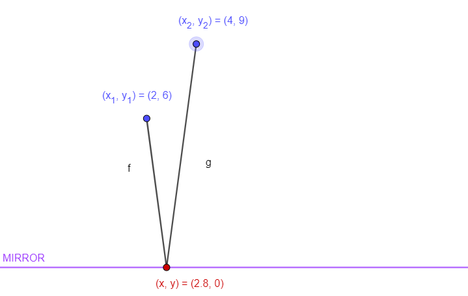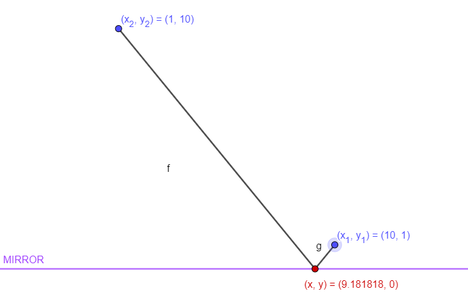# Practice on Toph

Participate in exhilarating programming contests, solve unique algorithm and data structure challenges and be a part of an awesome community.

# Magic Mirror on the Wall

By reborn · Limits 1s, 64 MB · Custom Checker

Kyablakanto and his friend Chalakanti went into the Mirror Room after browsing through the fair. In the Mirror Room, in front of the huge mirror, each of them waved to their reflections on the mirror. But, Kyablakanto being a little dumb, can't figure out where he needs to look on the mirror to see his friend. If he waves at wrong direction, he fears that she might leave him in the fair alone. That's why he called you. He knows you are an excellent programmer. As a friend, you have to help him.

You can consider the mirror to be infinite and compared to that Kyablakanto and Chalakanti seem like dots. On a 2-dimensional Cartesian coordinate system, the mirror is on the x-axis ($y = 0$ line) and Kyablakanto, Chalakanti are at $(x_1, y_1)$ and $(x_2, y_2)$, respectively. Your job is to find out a point $(x, y)$ on the mirror such that if Kyablakanto looks at that point, he can see his friend there.

## Input

The first line of input contains an integer $T$. You need to solve $T$ test cases.

Input follows $T$ lines. Each line corresponds to the input for one test case. Each of the line contains 4 space separated integers $x_1, ~y_1, ~x_2, ~y_2$.

### Constraints

• $1 \leq T \leq 10^5$.
• $1 \leq x_1, y_1, x_2, y_2 \leq 10^9$.
• $x_1 \neq x_2$.

• For 30 points: $y_1 = y_2$.
• For 70 points: $y_1$ can be different from $y_2$.

## Output

You need to output $T$ lines. Each line should contain two real numbers who represent $(x, y)$. $i$-th line should contain the answer to the $i$-th test case.

For every output number, if the absolute difference between your and judge's output are less than $10^{-4}$, your output will be considered correct. That means, if one of your output is $A$ and the judge has $B$ as it's output, your output would be considered correct if $| A - B | < 10^{-4}$ is satisfied.

## Sample

InputOutput
2
2 6 4 9
10 1 1 10

2.8000000000000000 0.0000000000000000
9.1818181818181818 0.0000000000000000


### Explanation of Sample IO

There are $T = 2$ test cases.

Following image should describe the first test case.The following picture describes the second test case.Geometry

### Statistics

89% Solution Ratio

aNkanpy.pritomEarliest, Jun '20

HKShakibFastest, 0.0s

Tahmid690Lightest, 1.4 MB

imamanik05Shortest, 83B

### Submit

 NHSPC 2020 PracticeJul '20 Replay of National High School Programming Contest 2020 - National RoundJul '20 National High School Programming Contest 2020 - National RoundJun '20 Sad Day To Die HardAug '20 Free Online Class-2020 Live - Demo ContestOct '20 Practice Contest Before IUTCPCDec '20